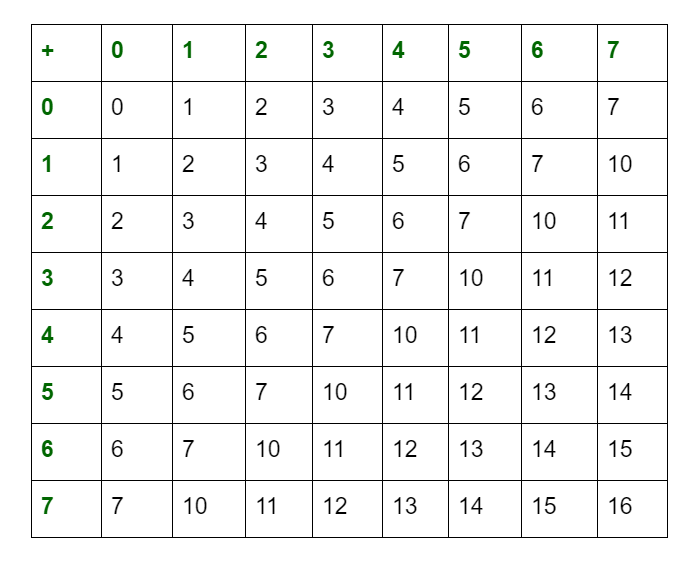# Arithmetic Operations of Octal Numbers

Generally, Octal Number representation is expressed by subscript 8 or octal (o). It has a base of 8 digits ranging from 0 to 7 (i.e., 0, 1, 2, 3, 4, 5, 6, 7). Arithmetic operations of octal numbers are also performed the same as decimal or binary arithmetic operations. The addition table for octal numbers is given as below:With the help of the above table, we can perform following arithmetic Operations of Octal Numbers :

It can perform additions of octal numbers.
2. Subtraction :
It can perform subtraction of octal numbers same as decimal number subtractions. But you can only borrow a number 7, which is the maximum digit allowed in octal number systems.
3. Multiplications :
If the product is less than radix of octal (i.e, 8), then we take it as the result, else divide it by radix of octal (i.e., 8) and take the remainder as the LSB (least significant bit). The quotient is taken as carry in the next significant digit.
4. Division :
It can be performed by following the rules of division of decimal numbers but the maximum allowed digit will be 7.

Attention reader! Don’t stop learning now. Get hold of all the important CS Theory concepts for SDE interviews with the CS Theory Course at a student-friendly price and become industry ready.

My Personal Notes arrow_drop_upIf you like GeeksforGeeks and would like to contribute, you can also write an article using contribute.geeksforgeeks.org or mail your article to contribute@geeksforgeeks.org. See your article appearing on the GeeksforGeeks main page and help other Geeks.

Please Improve this article if you find anything incorrect by clicking on the "Improve Article" button below.